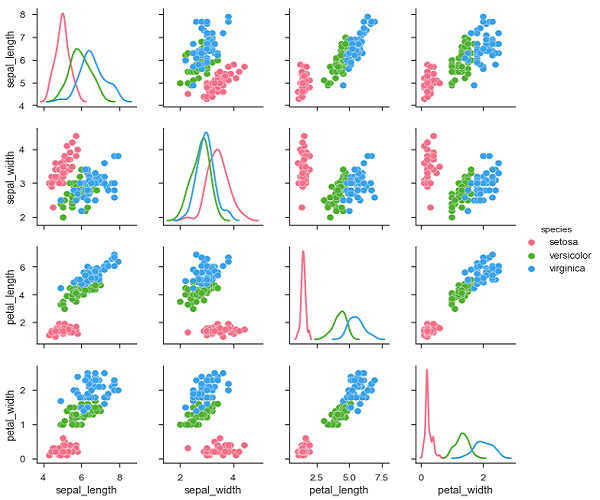Seaborn - Visualizing Pairwise Relationship

Datasets under real-time study contain many variables. In such cases, the relation between each and every variable should be analyzed. Plotting Bivariate Distribution for (n,2) combinations will be a very complex and time taking process.

To plot multiple pairwise bivariate distributions in a dataset, you can use the pairplot() function. This shows the relationship for (n,2) combination of variable in a DataFrame as a matrix of plots and the diagonal plots are the univariate plots.

Axes

In this section, we will learn what are Axes, their usage, parameters, and so on.

Usage

seaborn.pairplot(data,…)

Parameters

Following table lists down the parameters for Axes −

Sr.No. Parameter & Description
1

data

Dataframe

2

hue

Variable in data to map plot aspects to different colors.

3

palette

Set of colors for mapping the hue variable

4

kind

Kind of plot for the non-identity relationships. {‘scatter’, ‘reg’}

5

diag_kind

Kind of plot for the diagonal subplots. {‘hist’, ‘kde’}

Except data, all other parameters are optional. There are few other parameters which pairplot can accept. The above mentioned are often used params.

Example

import pandas as pd
import seaborn as sb
from matplotlib import pyplot as plt
sb.set_style("ticks")
sb.pairplot(df,hue = 'species',diag_kind = "kde",kind = "scatter",palette = "husl")
plt.show()

OutputWe can observe the variations in each plot. The plots are in matrix format where the row name represents x axis and column name represents the y axis.

The diagonal plots are kernel density plots where the other plots are scatter plots as mentioned.

Useful Video Courses

Video

Data Visualization using MatPlotLib & Seaborn

11 Lectures 4 hours

Video

Seaborn with Python

11 Lectures 2.5 hours